# Autocovariance

(diff) ← Older revision | Latest revision (diff) | Newer revision → (diff)
of a stochastic processThe covariance of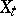and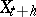. If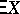denotes the mathematical expectation of a random variable, then the autocovariance equals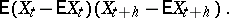The term "autocovariance" is usually applied to stationary (in the wide sense) stochastic processes (cf. Stationary stochastic process). For such processes the autocovariance depends only on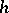and differs from the auto-correlation by the presence of a single factor which is equal to the variance of. The terms "covariance function" and "autocovariance function" are used together with the term "autocovariance" .